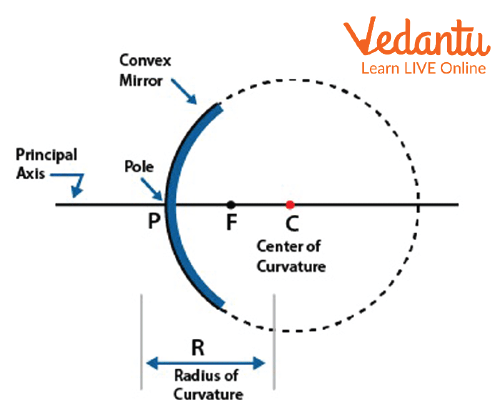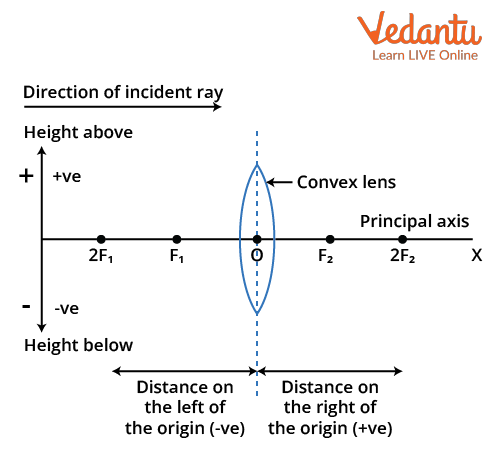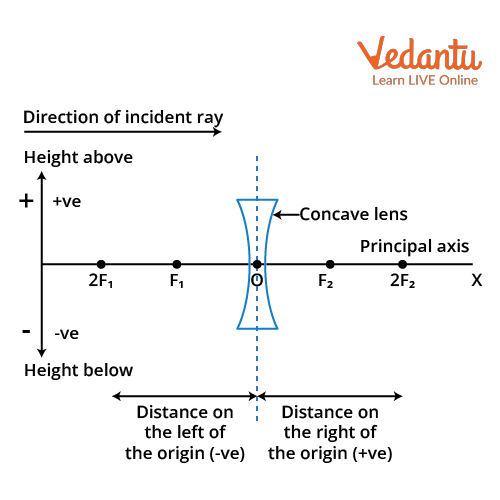Courses
Courses for Kids
Free study material
Free LIVE classes
More

# Sign Convention in Optics - JEE Important TopicLIVE
Join Vedantu’s FREE Mastercalss

## What is a Sign Convention?

The sign convention is the set of rules and regulations which is required to follow for the proper analysis of optics. These sign convention rules are very useful for the complex problem related to the lens formula and mirror formula.

In this article, the sign convention for mirror and lens, sign convention for the concave and convex mirror, sign convention for spherical lenses, spherical mirror, and the new Cartesian sign convention for lens have been explained briefly.

## Sign Convention for Spherical Mirror

It is known that a spherical mirror is of two types, i.e. concave mirror and convex mirror. As the configuration of both mirrors is different from each other, hence their sign conventions are also different. The sign convention for both the mirror is given below:

## Sign Convention for Concave Mirror and Convex Mirror

The concave mirror is a mirror that has a reflective surface on the inwardly curved surface of the spherical mirror. The image for the same is shown below (in image 1). The convex mirror is a mirror that has a reflective surface on the outward curved surface of the spherical mirror. The image for the same is shown below (in image 2).Concave mirrorConvex mirror

According to the image of the concave mirror (image 1) and convex mirror (image 2), the mirror has a centre of curvature C, focus F, and pole, P. A line which passes through the centre of curvature, focus, and the pole is called the principal axis. The point at which the principal axis meets the mirror is called a pole.

## Sign Convention

• All the distances which were measured for the ray and calculation will be taken from the pole of the mirror.

• All the measurements which were done in the direction of the incident ray (like in this case, from left to right) are taken as positive, and against the direction of the incident ray is negative. In the given cases, the focal length for the concave mirror is negative and the focal length for the convex mirror is positive.

• All the measurements taken above the principal axis are positive, while those below the principal axis are negative.

• An object is always placed to the left of the mirror. The object distance measured from the pole will be against the direction of the incident ray, hence, it will be negative.

## Sign Convention for Spherical Lens

It is known that spherical lenses are of two types, i.e. concave lens and convex lens. Hence, the configuration of both lenses is different from each other. Therefore, their sign conventions are also different. The sign convention for both the lenses are given below:

### 1. Sign convention for Convex Lens and Concave Lens:

A convex lens is a lens that is made up of two spherical surfaces that are bent outward in configuration. Such lenses are also known as biconvex lenses. This lens converges all the parallel beams coming towards it, passes through the lens, and focuses all of them at the focus of the convex lens. The image of the convex lens is shown below (in image 3).A convex lens

A concave lens is a lens that is made up of two spherical surfaces that are bent inwards in configuration. Such lenses are also known as biconcave lenses. This lens diverges all the parallel beams coming towards it, passes through the lens, and diverges all of them. The image of the concave lens is shown below (in image 4).A Concave lens

According to the image of the convex lens and concave, it has been seen that the lens has an optical centre at O, two foci F1 and F2, and a centre of curvature of the lens 2F1 and 2F2. A line that passes through the centre of curvature, foci, and optical centre is called the principal axis. The point at which the principal axis meets the lens is called the optical centre.

## Sign Convention

• All the distances which were measured for the ray and calculation will be taken from the optical centre of the lens.

• All the measurements which were done in the direction of the incident ray (like in this case from left to right) are taken as positive and against the direction of the incident ray is negative. In the given cases, the focal length of a convex lens is positive and the focal length of a concave lens is negative.

• All the measurements taken above the principal axis are positive while below the principal axis are negative.

• An object is always placed to the left of the lens. The object distance measured from the optical centre will be against the direction of the incident ray, hence, it will be negative.

## New Cartesian Sign Convention

According to the new Cartesian sign for the lens, there is some sign convention that is required to follow for the refraction of the light. These are given below:

• All the distances which were measured for the ray and calculation will be taken from the optical centre of the lens.

• All the measurements which were taken in the direction of the incident ray (like in this case from left to right) are taken as positive and against the direction of the incident ray are negative. In the case of a convex lens, the focal length is positive while it is negative in a concave lens.

• All the measurement of height taken above the principal axis is positive while below the principal axis is negative.

## Conclusion

This article concludes with brief information about the sign convention rules of the mirror and lens. It explains each aspect of the sign convention for a lens with the help of respective diagrams. It has discussed the sign convention for mirror and lens, and the sign convention for concave and convex mirror in a simple way. It has also shared a glimpse of the new Cartesian sign convention for the convex and concave lens. It has also concluded various remarks about the sign convention of the lens in case of refraction of light.

Last updated date: 22nd Sep 2023
Total views: 133.5k
Views today: 3.33k

## FAQs on Sign Convention in Optics - JEE Important Topic

1. Why is the sign convention important in optics while working on mirrors and lenses?

Sign convention has very high importance while working with mirrors and lenses as it has a defined set of proper rules for image formation analysis. Sign convention identifies the type of the image whether it is virtual or real and the orientation of the image whether it is inverted or erect. The sign convention helps for the determination of the magnification of the mirror. Various parameters will be determined based upon the sign convention given as focal length, distance and orientation of object, distance, type and orientation of the image formed after reflection through mirror and refraction through the lens.

2. Explain the importance of optics in real life.

Optics is a vital part of our everyday life. Various types of visual systems require the concept of optics for their work in a proper manner. Optical devices like cameras, telescopes and binoculars work on the concept of lenses and mirrors for the functioning of the devices. Lenses are used in the eyepiece of the microscope for enlarging small objects. Plane mirrors are used for personal use in everyday life. Similarly, lenses are used in spectacles and contact lenses for human beings suffering from eye-sight problems.

3. What is the weightage of optics in the JEE exam?

Optics carry high weightage in competitive exams like . It involves many concepts relating to ray optics, a reflection of light, refraction of light through glass slabs and most importantly the numerical relation to the concept of mirror formula and lens formula. It has also other important concepts like dispersion of light, and prism which have importance according to the JEE Main point of view. In JEE Main 2022 exam a total of 3 questions were asked about optics thus optics had a weightage of 6.6% in  JEE Main 2022.